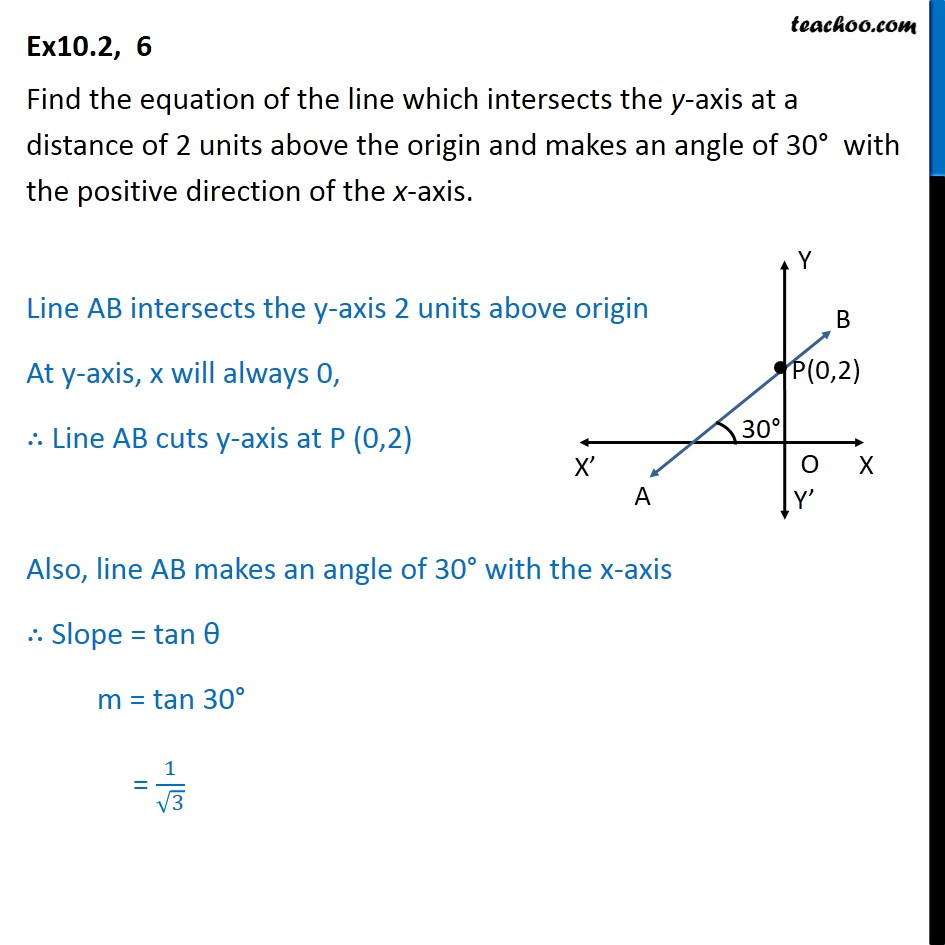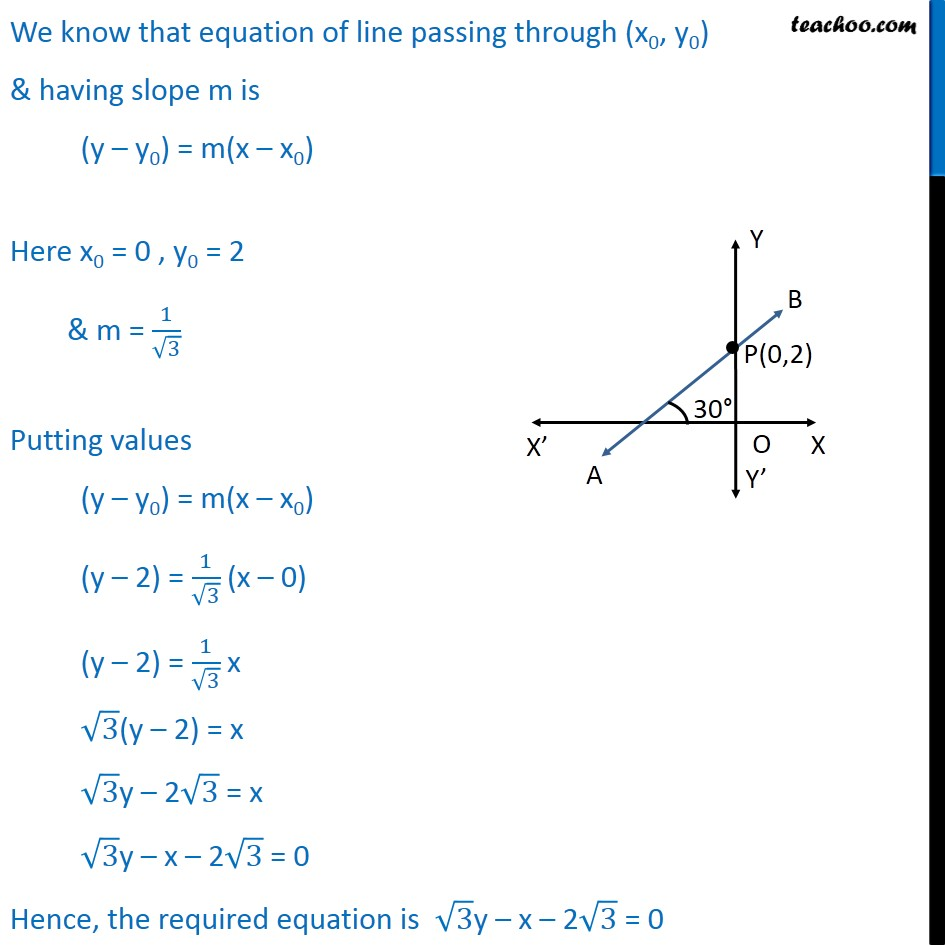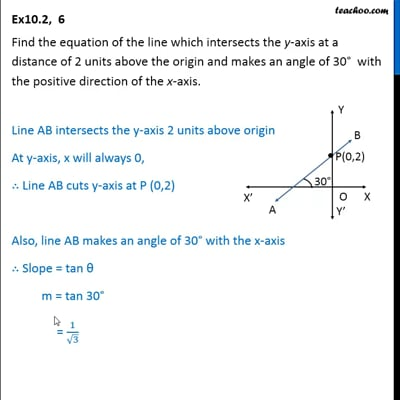Ex 10.2

Chapter 10 Class 11 Straight Lines
Serial order wiseThis video is only available for Teachoo black users

Solve all your doubts with Teachoo Black (new monthly pack available now!)

### Transcript

Ex10.2, 6 Find the equation of the line which intersects the y-axis at a distance of 2 units above the origin and makes an angle of 30° with the positive direction of the x-axis. Line AB intersects the y-axis 2 units above origin At y-axis, x will always 0, ∴ Line AB cuts y-axis at P (0,2) Also, line AB makes an angle of 30° with the x-axis ∴ Slope = tan θ m = tan 30° = 1/√3 We know that equation of line passing through (x0, y0) & having slope m is (y – y0) = m(x – x0) Here x0 = 0 , y0 = 2 & m = 1/√3 Putting values (y – y0) = m(x – x0) (y – 2) = 1/√3 (x – 0) (y – 2) = 1/√3 x √3(y – 2) = x √3y – 2√3 = x √3y – x – 2√3 = 0 Hence, the required equation is √3y – x – 2√3 = 0